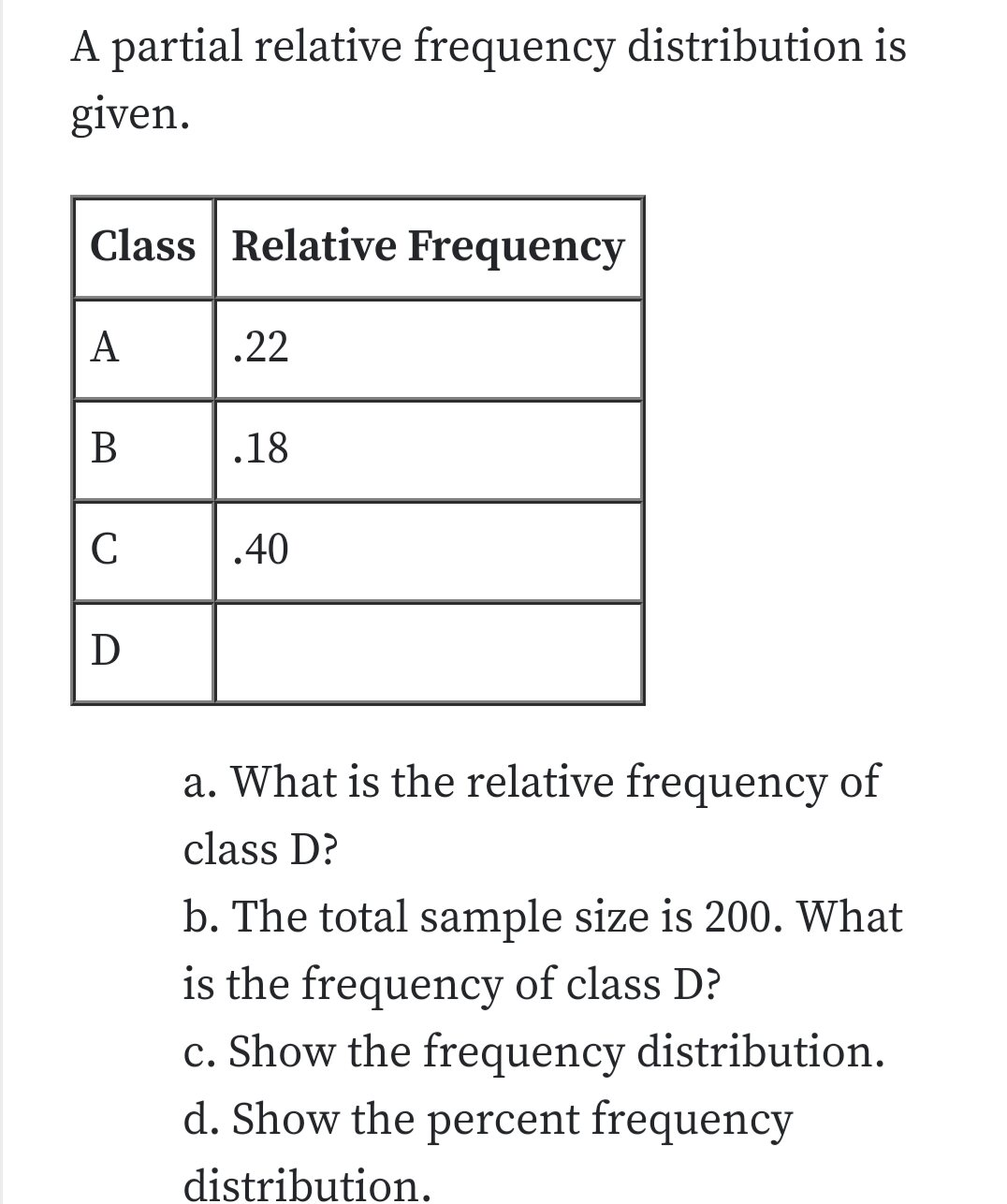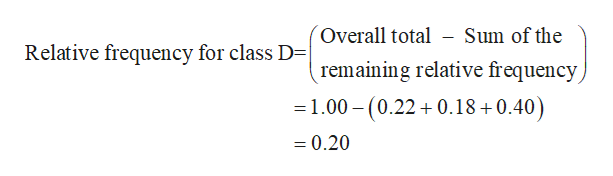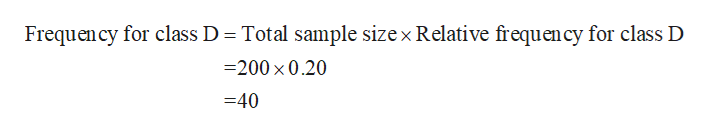# A partial relative frequency distribution isgivenClass Relative FrequencyA.22В.18С40a. What is the relative frequencyofclass D?b. The total sample size is 200. Whatis the frequency of class D?c. Show the frequency distributiond. Show the percent frequencydistribution

Question
69 viewshelp_outlineImage TranscriptioncloseA partial relative frequency distribution is given Class Relative Frequency A .22 В .18 С 40 a. What is the relative frequency of class D? b. The total sample size is 200. What is the frequency of class D? c. Show the frequency distribution d. Show the percent frequency distribution fullscreen
check_circle

Step 1

Hey there! Thank you for posting the question. Since your question has more than 3 parts, we are solving the first 3 parts for you, according to our policy. If you need help with any of the other parts, please re-post the question and mention the part you want answered.

Step 2

a)

Relative frequency distribution:

Relative frequency distribution is obtained by dividing the respective frequencies of each class by the total number of observations in the study. The overall total of the relative frequency is about 1.00.help_outlineImage TranscriptioncloseOverall total - Sum of the remaining relative frequency 1.00 (0.220.18+0.40 Relative frequency for class D-| =0.20 fullscreen
Step 3

b)

Let n be the total sample size (n=200) and the relativ...help_outlineImage TranscriptioncloseFrequency for class D Total sample sizex Relative frequency for class D -200 x 0.20 =40 fullscreen

### Want to see the full answer?

See Solution

#### Want to see this answer and more?

Solutions are written by subject experts who are available 24/7. Questions are typically answered within 1 hour.*

See Solution
*Response times may vary by subject and question.
Tagged in
MathStatistics